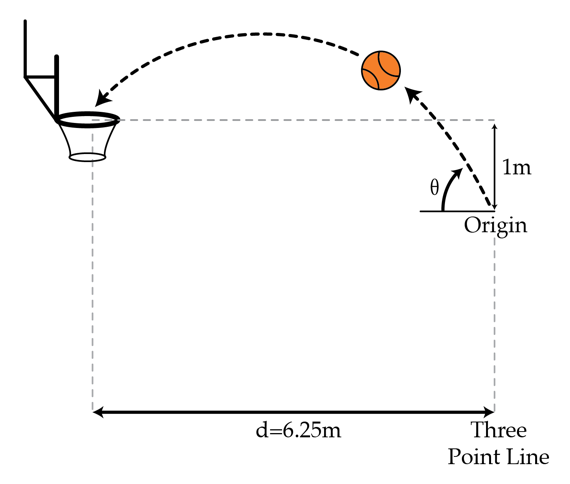# Calculations like this are why NBA players take so long to shoot free throws

An NBA basketball player want to score 3 points, so he shoots the ball right before the 3-point-line (which is $d=6.25~\mbox{m}$ horizontally away from the basket). The difference in height from where he releases the ball and the basket is roughly $h=1~\mbox{m}$. Given the gravitational acceleration $a_g=-9.8~\mbox{m/s}^2$, at what angle to the horizontal in degrees did he shoot the ball if the horizontal speed of the ball is $8.71~\mbox{m/s}$?Details and assumptions

• You may neglect air resistance.
×

Problem Loading...

Note Loading...

Set Loading...